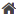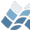NV5 GEOSPATIAL SOFTWARE>  Docs Center  >  Libraries  >  Mankoff  >  KDM_ARRSETSIZEKDM_ARRSETSIZE

KDM_ARRSETSIZE

## Purpose

This function resizes a 1D array. It can truncate or expand
the array. When expanding it can optionally fill the new
elements with a default value.

Array

## Calling Sequence

Result = KDM_ARRSETSIZE( Array, newSize )

## Inputs

Array: An array of any type up to 8 dimensions
NewSize: The new length of the array. Each dimension can be
shorter, longer, or equal to the original length.

## Keyword Parameters

_EXTRA=e is passed to MAKE_ARRAY (VALUE might be useful)

## Outputs

A new array, based on the input array. For each dimension of the
array, if newSize[dim] is shorter then the result contains the
values in the original array dimension up to the truncation
point. If newSize is equal to the original array dimension length
then the new array is the same as the input array. If newSize is
larger than the length of the original array then the new array is
longer, filled with zero by default, or the value specified by the
VALUE keyword passed to MAKE_ARRAY.

## Restrictions

I haven't tested it with arrays of structures or pointers yet.

## Procedure

For 1D array, the algorithm is the following.
n = n_elements(arr)
if len eq n then return, arr ; new size eq current size
if len lt n then return, arr[0:len-1] ; new size lt current size
arr2 = MAKE_ARRAY( len, TYPE=SIZE(arr,/TYPE), _EXTRA=e )
arr2[ 0:n-1 ] = arr
return, arr2
The code works for arrays up to 8 dimensions due to IDL allowing
you to subscript non-existent dimensions with  or [0:0], such
as:
x = indgen(3)
print, x[ 0:2, 0:0, 0:0 ]

## Example

x = indgen(3)
;; shorten X to 2 elements
print, kdm_arrsetsize( x, 2 )
;; grow X to 5 elements
print, kdm_arrsetsize( x, 5 )
;; grow X to 5 elements and use a default value
print, kdm_arrsetsize( x, 5, VALUE=42 )
;; Trim a 5x5 array to a 3x2 array
print, kdm_arrsetsize( bindgen(5,5), [3,2] )
;; Works with strings too. Take an array of 2 and expand it to 4,
;; filling with a default value of 'Goodbye'
print, kdm_arrsetsize( ['hello','world'], 4, value='Goodbye' )

## Modification History

Written by: Ken Mankoff
2010-03-05: KDM. Added documentation. Expanded to support 8 dimensions.

© 2023 NV5 Geospatial |  Legal